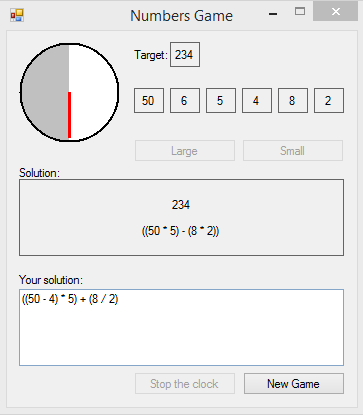#Numbers Game

## Overview

This is the Numbers Game as played on the UK Channel Four tv game show CountDown. The game is simple, you select 6 random numbers, and attempt to reach a random target by adding, subtracting, multiplying, and dividing those numbers.

The numbers used are four large numbers... 100, 75, 50, 25, and twenty small numbers... two each of the numbers 1 to 10.

You can randomly choose from 0 to 4 large numbers, with the remaining numbers being small numbers. When you select your sixth random number, a random target number will be chosen (from 101 to 999), the countdown clock will begin, and you have thirty seconds to find a solution that equals the target number. Your solution must consist of any of your chosen numbers, the arithmetic operators +, -, /, *, and brackets. You only need to use as many of the six numbers as you need. It's not obligatory to use all of the numbers.

During the thirty-second countdown, the application will attempt to solve the equation. If a solution exists, it will be found within the thirty-second duration (Exceptional cases might take slightly longer). If you think you've found a solution, you can stop the clock and the PC solution will appear as soon as it is available.

This is a difficult game to beat, but it is possible if you're quick with mental arithmetic.Figure 1 - The game at runtime.

## The Numbers Class

This holds the numbers used in the game. Each new game the Integer Lists large and small are reloaded with the large numbers {25, 50, 75, 100}, and the small numbers 2 each of numbers (1 to 10). These Lists are then randomly shuffled.

When the user requests a large number, a number is chosen then removed from the large List. The same applies when requesting a small number.

As both Lists are already shuffled randomly, the first number in the List is chosen.

```Public Class Numbers

Private large As New List(Of Integer) From {25, 50, 75, 100}
Private small As New List(Of Integer) From {1, 2, 3, 4, 5,
6, 7, 8, 9, 10,
1, 2, 3, 4, 5,
6, 7, 8, 9, 10}

Public Sub New(r As Random)
large = large.OrderBy(Function(x) r.NextDouble).ToList
small = small.OrderBy(Function(x) r.NextDouble).ToList
End Sub

Public Function drawSmall() As Integer
Dim x As Integer = small(0)
small.RemoveAt(0)
Return x
End Function

Public Function drawLarge() As LargeDrawn
Dim x As Integer = large(0)
large.RemoveAt(0)
Dim isLastNumber As Boolean = large.Count = 0
Return New LargeDrawn With {.x = x, .last = isLastNumber}
End Function

End Class
```

## The Graphical Countdown ClockFigure 2 - The Countdown Clock.

The game lasts for 30 seconds, from when the user selects the last random number, until the game is over.
Hopefully, by this time both the user has their solution, and the solver has found a solution. Most times, the solver will find a solution within the 30 seconds.
On reaching a solution, the user can stop the clock early, and the solution found by the solver will be displayed as soon as it is available.

## The CompositeNumber Class

The solver is a brute force solver. It tries all possible combinations of the 6 chosen random numbers. In Figure 1, the numbers 50 and 5 are multiplied to make the composite number 250. Also the numbers 8 and 2 are multiplied to make the composite number 16. These 2 composite numbers are then subtracted to make the composite number 234, which is the correct solution.

This class holds the numbers, operators, and textual equation string which make this composite number.

```''' <summary>
''' Contains the numbers and operators used to make a Composite Number
''' Builds the bracketed tostring used in displaying the solution
''' </summary>
Public Class CompositeNumber

Private equationString As String
Private arrayNumbers() As Integer
Private arrayOperators() As String
Public Sub New(Numbers() As Integer, Operators() As String, equationStrings() As String, operatorIndex As Integer)
Me.arrayNumbers = Numbers
Me.arrayOperators = Operators
If equationStrings.Length = 0 Then
Me.equationString = Numbers(0).ToString
ElseIf equationStrings.Length = 2 Then
Me.equationString = "(" & equationStrings(0) & " " & Operators(operatorIndex) & " " & equationStrings(1) & ")"
End If
End Sub

Public Function getNumbers() As Integer()
Return Me.arrayNumbers
End Function

Public Function getOperators() As String()
Return Me.arrayOperators
End Function

Public Overrides Function tostring() As String
Return Me.equationString
End Function

End Class
```

The chosen numbers, then the composite numbers they make up are run through the solver several times to find the closest solution.

Computers are ideal for manipulating numbers. An advanced arithmetical number manipulating program is only as good as the instructions it is given, although advances in AI technology might change that in the future...

You can download the example project here...